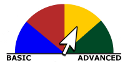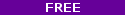# Differential Equations in ActionIn this course you will examine real world problems -- rescue the Apollo 13 astronauts, stop the spread of epidemics, and fight forest fires -- involving differential equations and figure out how to solve them using numerical methods. You'll need a basic knowledge of programming for this course, around the level of CS 101 or equivalent. You'll also need to understand trigonometry at the high school level, as well as basic vector algebra. This class will primarily involve solving equations numerically rather than analytically, but some exposure to calculus and physics at the level of PH 100 wouldn't hurt. By the end of this course, you'll develop an intuition for the use of differential equations in the applied sciences. You'll also learn how to build mathematical models for systems of differential equations. Along the way, you'll learn how to translate mathematical expressions into Python code, and solve some really cool problems!